Download PresentationSoftware Metrics for Object Oriented Design

Loading in 2 Seconds...

# Software Metrics for Object Oriented Design - PowerPoint PPT Presentation

Software Metrics for Object Oriented Design. C K and M OOD Metrics. Eric Gitangu Manali Patel. O utline. Definition and Use of Software Metrics. CK and Mood Metrics with Examples Conclusion. U se of S oftware M etrics.I am the owner, or an agent authorized to act on behalf of the owner, of the copyrighted work described.
Download Presentation## Software Metrics for Object Oriented Design

An Image/Link below is provided (as is) to download presentation

Download Policy: Content on the Website is provided to you AS IS for your information and personal use and may not be sold / licensed / shared on other websites without getting consent from its author.While downloading, if for some reason you are not able to download a presentation, the publisher may have deleted the file from their server.

- - - - - - - - - - - - - - - - - - - - - - - - - - E N D - - - - - - - - - - - - - - - - - - - - - - - - - -
Presentation Transcript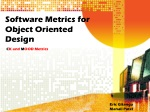### Software Metrics for Object Oriented Design

CK and MOOD Metrics

Eric Gitangu

Manali Patel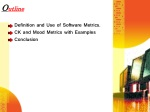Outline

Definition and Use of Software Metrics.

CK and Mood Metrics with Examples

Conclusion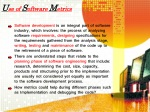Use of Software Metrics

Software development is an integral part of software industry, which involves: the process of analyzing software requirements, designing specifications for the requirements gathered from the analysis stage, writing, testing and maintenance of the code up to the retirement of a piece of software.

There are understated steps that relate to the planningphase of software engineering that include: research, determining the cost, size, capacity, products and structuring prior to the implementation are usually not considered yet equally as important to the software development process.

How metrics could help during different phases such as Implementation and debugging the code?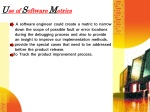Use of Software Metrics

• A software engineer could create a metric to narrow
• down the scope of possible fault or error locations
• during the debugging process and also to provide
• an insight to improve our implementation methods.
• provide the special cases that need to be addressed
• before the product release.
• To Track the product improvement process.Software Metrics

As an aid to the software industry, software metrics have been introduced to help improve the implementation process, testing and maintenance of the product.

A Software Metric offers a quantitative approach to measure a particular property of a piece of Software.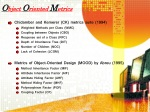Object Oriented Metrics
• Chidamber and Kemerer (CK) metrics suite (1994)
• Weighted Methods per Class (WMC)
• Coupling between Objects (CBO)
• Response set of a Class (RFC)
• Depth of Inheritance Tree (DIT)
• Number of Children (NOC)
• Lack of Cohesion (LCOM)
• Metrics of Object-Oriented Design (MOOD) by Abreu (1995)
• Method Inheritance Factor (MIF)
• Attribute Inheritance Factor (AIF)
• Attribute Hiding Factor (AHF)
• Method Hiding Factor (MHF)
• Polymorphism Factor (POF)
• Coupling Factor (CF)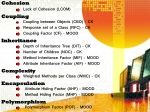Cohesion

• Lack of Cohesion (LCOM)

Coupling

• Coupling between Objects (CBO) - CK
• Response set of a Class (RFC) - CK
• Coupling Factor (CF) - MOOD

Inheritance

• Depth of Inheritance Tree (DIT) - CK
• Number of Children (NOC) - CK
• Method Inheritance Factor (MIF) - MOOD
• Attribute Inheritance Factor (AIF) - MOOD

Complexity

• Weighted Methods per Class (WMC) - CK

Encapsulation

• Attribute Hiding Factor (AHF) - MOOD
• Method Hiding Factor (MHF) - MOOD

Polymorphism

• Polymorphism Factor (POF) - MOOD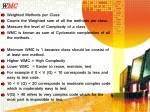WMC
• Weighted Methods per Class
• Counts the Weighted sum of all the methods per class.
• Measure the level of Complexity of a class
• WMC is known as sum of Cyclomatic complexities of all the methods .
• Minimum WMC is 1 because class should be consist of at least one method.
• Higher WMC = High Complexity
• Lower WMC = Easier to reuse, maintain, test
• For example if 0 < V (G) < 10 corresponds to less and easy to test code.
• 10 < V (G) < 20 corresponds to moderate complex code which is moderately easy to test.
• V(G) > 40 refers to very complex code which for the most part is impossible to test.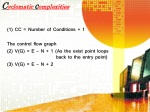Cyclomaticcomplexities

(1) CC = Number of Conditions + 1

The control flow graph

(2) V(G) = E – N + 1 (As the exist point loops back to the entry point)

(3) V(G) = E – N + 2WMC - To Calculate Cyclomatic Complexities

Each method has base complexity as 1

IF….Else with single decision = +1

if (c>3 || c < 20) = +2 because 2 conditions

Case = +1

While loop = +1

// V(G) = +1

if( r1() ) { //V(G) = +2

m1(); }

else {

m2(); }

if( r2() ) { //V(G) = +3

m3(); }

else {

m4(); }WMC - To Calculate Cyclomatic Complexities

V(G) = 9E – 7N + 1 V(G) = 9E – 8N + 2

= 3 = 3DIT
• Depth of Inheritance Tree
• Calculates the length/depth of inheritance level from the class to the inheritance tree i.e. the longest path from the class to its root.
• The DIT is the count of the level of subclasses effecting a class.
• The value 0 of the DIT indicates a root with no subclasses.
• High DIT -> deeper inheritance -> the complexity of the class becomes greater -> increases the number of faults as more methods and variable have been reused leading to increased coupling.DIT Example

DIT level of subclass 4 and 5 is = 2NOC
• Number of Children (NOC)
• counts the number of subclasses derived from a class and measures the breadth of the class.
• NOC is counting the number of times the parent class methods have been inherited by subclasses.
• Increased NOC ->indicates higher modularity -> reduces the probability of errors arising and also promotes reusability since with higher modularity we may be able to debug individual modules separately hence a lower probability of error detection.NOC Example

There are 9 classes that inherits the class A and thereforeNOC= 9MIF
• Method Inheritance factor
• MIF = inherited methods/total methods available in classes i.e. the ratio of inherited methods to total number methods of available classes.
• Both MIF and AIF should be maintained at mediocre ratios since too high a ratio of either indicates excessive inheritance and too low a ratio indicates a poor object-oriented framework.#include <iostream> MIF = inherited methods/ total methods

using namespace std; = 2/3 = 0.6

class A {

public:

A() { a_member = 0; }

protected:

int a_member;

class B{

public:

B() { b_member = 0; }

protected:

int b_member; };

class C : public A, public B {

public:

C() : A(), B() { c_member = 0; }

protected:

int c_member; };AIF
• Attribute Inheritance Factor
• AIF = inherited attributes/total attributes available in classes i.e. the ratio of inherited attributes to the total number of attributes.class Polygon {

protected: int width, height, x, y; //4

public: void setParameters (int w, int h);

{

width = w;

height = h;

} };

class Rectangle : public Polygon //inheriting from Polygon {

public: int calculateArea ()

{ return (width*height); } }; // inheriting 2 attributes

int main ()

{ ….}

AIF = inherited attributes/total attributes available in classes

= 2/4

= 0.5Coupling – CBO

RFC

CF

By EricCBO
• Coupling Between Objects
• counts the number of classes coupled with a considered class.
• High coupling between classes -> increases the complexity of the class and makes it difficult to reuse and test the program. It is also difficult to maintain and increases the number of errors/faults since the larger number of coupling requires more time and deeper understanding while making changes to improve the product. Therefore, the object coupling between classes should be minimum.
• Coupling considerably increases when we have methods of one class calling or using attributes associated with another class.CBO Example

Class F has the coupling relation with class A,B,C and E CBO = 4

public class Class F extends Class E implements Serializable

{

ClassBobjB;

public Class C foo( ClassAobjA) throws Exception{

int noConstants = Constant.MAX_VAL;

try(objB.isGreaterThan(objA)) {

noConstants = objA .getValue(); }

Catch (Exception ex) {ex.stacktrace();}

return new ClassC ();RFC
• Response set of  a Class
• It is the set of methods that are invoked in the response of a message to a class.
• As the number of outcomes increases, the class’ complexity becomes higher.
• For example, when we are dynamically allocating new objects within a thread of execution we may create numerous objects within the thread, the RFC would determine the total number of outcomes that would evolve from execution of the thread or an instance of a class. As the result, RFC becomes higher and as the understanding of the interaction between objects becomes harder, the testing and debugging problem increases.RFC Example

Class Pump {

void firstmethod()

{

secondmethod():

}

void secondmethod()

{

thirdmethod():

}

void thirdmethod()

{

System.Out.Println(“We called 3 methods on response to new pump”);

}

Public Static void main(String [] args)

{

Pump mypump = new Pump();

mypump.firstmethod();

}

}

RFC = 4 (including println)CF
• Coupling Factor
• It is the ratio of actual coupling vs. maximum number of potential coupling in a class
• It is measured as percentage from 0% - 100%. We ideally thrive for low coupling i.e. independence coupling.
• In computational terms we thrive for a CF level of <=10% out of a maximum 100%.CF Example

#include <iostream>

using namespace std;

class A {

public:

A() { a_member = 0; }

protected:

int a_member;

class B{

public:

B() { b_member = 0; }

protected:

int b_member; };

class C : public A, public B {

public:

C() : A(), B() { c_member = 0; } //Calling methods of another class

protected:

int c_member; };

CF = actual coupling / maximum number of potential coupling among classes

= 2/10 = 20%LCOM
• LCOM measure the level of cohesion and is used to determine the lack of cohesion.
• It is about measuring the number of the pair methods that have shared the at least one(Q) or different set of variables in a class(P).
• Based on the pair methods, we increase P or Q by one where
• LCOM = P - Q, if P> Q
• otherwise LCOM = 0.
• The class is considered to be highly cohesive if LCOM = 0 and if  LCOM > 0, then a class is not well cohesive and the higher value of LCOM can result into fault or error.LCOM Example 1

public class A { To measure LCOM

private int f2; Compare two methods

Private int f3; if share variable increase Q

Private int f4; if no share variable increase P

Private int f5;

public void method1() {

// Uses f1; method 1 & method 2 -> Q = 1

// Uses f2; } method 1 & method 3 -> P = 1

public void method2() { method 2 & method 3 -> P = 2

// Uses f2;

// Uses f3; } LCOM = P-Q if P>Q

public void method3() { LCOM = 1

// Uses f4;

// Uses f5; }LCOM Example 2

LCOM = 8 LCOM = 8

P = 9 P = 18

Q = 1 Q = 10MHF
• Method Hiding Factor
• It is a fraction in which the denominator is the number of total methods whereas the numerator is the total of encapsulated methods defined in all the classes.
• If all methods are private/protected, MHF = 100%, High encapsulation decreases the complexity since encapsulated methods dictate the scope from which they may be accessed therefore limiting the number of locations which makes the debugging process easier.
• If all methods are public, MHF = 0% shows methods are unprotected and chances of errors are high.MHF Example

• class Polygon {
• protected: int width, height;
• public: void setParameters (int w, int h);
• {
• width = w;
• height = h;
• } };
• class Rectangle : public Polygon {
• public: int calculateArea ()
• { return (width*height); } };
• class Triangle : public Polygon {
• public int calculateArea ()
• { return( (width*height)/2); } };

MHF = the total of encapsulated methods/total methods

= 0AHF
• Attribute Hiding Factor
• It measures the total number of attributes encapsulated in the class.
• The AHF  may be expressed as a fraction in which the denominator is the number of total attributes whereas the numerator is the total of encapsulated attributes defined in all the classes.
• If all attributes are private/protected – MHF = 100%.
• If all attributes are public – MHF = 0% shows methods are unprotected and chances of errors are high.
• Same as MHFAHF Example

#include <iostream>

using namespace std;

class A {

public:

A() { a_member = 0; }

protected:

int a_member;

class B{

public:

B() { b_member = 0; }

protected:

int b_member; };

class C : public A, public B {

public:

C() : A(), B() { c_member = 0; }

public:

int c_member; };

AHF = the total of encapsulated attributes/ total attributes

= 2/3 = .6667PF
• Polymorphism Factor
• It is the ratio of number of overridden methods in the class vs the maximum number of methods that can be overridden.
• POF becomes higher with more overriding and the increases the level of complexity and lower with less overridden to the base class.
• POF is expressed as a percentage between 0% - 100%, as aforesaid, when PF=100%, all methods are overridden in all derived classes and correlates to a complex program.
• A PF value of 0% may indicates that a project uses no inheritance, polymorphism.
• We ideally want the PF value to range between 40% - 60% this would indicate a moderately complex program which correlates to a moderately hard program to test and or maintain.class Animal {

public:

virtual void eat() { std::cout << "I eat like a generic animal.\n"; }

virtual ~Animal() { }

};

class Wolf : public Animal {

public:

void eat() { std::cout << "I eat like a wolf!\n"; }

void tasteWolf() {}

};

class Fish : public Animal {

public:

void eat() { std::cout << "I eat like a fish!\n";}

void tasteFish() {}

};

PF = number of overridden methods/the maximum number of methods

that can be overridden.

= 1/3 = 0.3333Conslusion
• The object oriented paradigm is increasingly being used in the software industry such as Java, C++, C#, .NET Framework etc.
• The increased demand for Object-Oriented designs has necessitated the need for the adoption of well-defined structures that the CK and MOOD suite provides. CK and MOOD provide a medium through which software engineers can build robust, easy to maintain, relatively cheaper and more importantly provide a quantitative way of measuring the success of a project.Kaur, Amandeep, Satwinder Singh, Dr. K. S. Kahlon, and Dr. ParvinderSandhu. "Empirical Analysis of CK & MOOD Metric Suit.“ Ed. International Journal of Innovation, Management and Technology. Vol. 1December 5,2010. 1-6. Print.

Shaik, Amjan, C.R.K Reddy, BalaManda, Prakashini C., and Deepthi K. "Metrics for Object Oriented Design Software Systems: A Survey.” Ed. Journal of Emerging Trends in Engineering and Applied SciencesScholarlink Research Institute Journals, 2010. 1-9. Print.Questions?

Comments?